### Math Scanner By Photo Solve My Math Problem Premium V 7.1 APK

Developer :
Pic Frame Photo Collage Maker & Picture Editor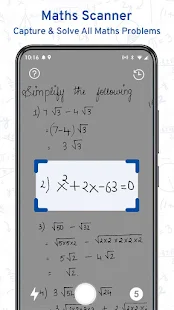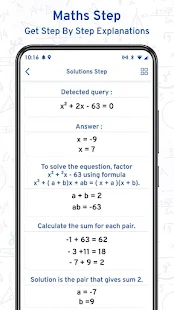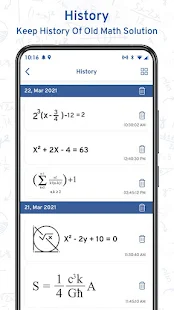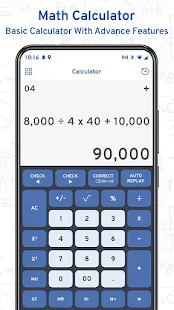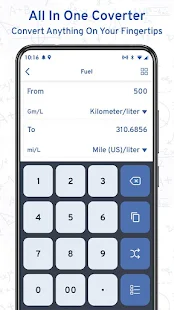Descriptions :

Math Scanner by Photo Solve my math problem Maths will give you the answer to any math question simply by taking a photo. With millions of users and many problems solved, math way is the world's smartest math calculator app. With a smart calculator, you just need to enter the formula and the equation calculator will give you the answers automatically. Just point your camera at a problem and the photo calculator will magically display the result with detailed step-by-step instructions. The calculator will give you the answer to any math question simply by taking a photo. The calculator is a great way to check your homework, study, and learn math.

Features:
- Calculate mathematical problems with the camera.
- Mathematical scanner without the need for the Internet.
- Step-by-step solutions for easy learning.
- Smart calculator with graphs and tables.
- Can solve simple to advanced math questions.
- You can solve addition, subtraction, multiplication, division, algebra, percentage, EMI, interest, etc.
- Click on a photo of any math problem and solve it for you.

Required Android Versions : Lollipop [5.0–5.0.2] - Marshmallow [6.0 - 6.0.1] - Nougat [7.0 – 7.1.1] - Oreo [8.0-8.1]